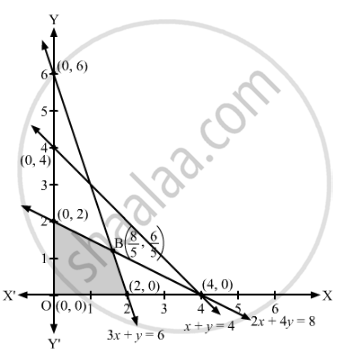# Find Graphically, the Maximum Value of Z = 2x + 5y, Subject to Constraints Given Below: 2x + 4y ≤ 8 3x + Y ≤ 6 X + Y ≤ 4 X ≥ 0, Y ≥ 0 - Mathematics

Find graphically, the maximum value of Z = 2x + 5y, subject to constraints given below:

2x + 4y ≤ 8
3x + y ≤ 6
x + y ≤ 4
x ≥ 0, ≥ 0

#### Solution

The given linear programming problem is Maximize Z = 2x + 5y

subject to the constraints

2x + 4y ≤ 8

3x + y ≤ 6

x + y ≤ 4

x ≥ 0, ≥ 0

Converting the inequations into equations, we obtain the following equations of straight lines:

2x + 4y = 8, 3x + y = 6, x + y = 4

The line 2x + 4y = 8 meets the coordinate axes at (4, 0) and (0, 2).

The line 3x + y = 6 meets the coordinate axes at (2, 0) and (0, 6).

The line x + y = 4 meets the coordinate axes at (4, 0) and (0, 4).

The feasible region determined by the given constraints can be diagrammatically represented as,The coordinates of the corner points of the feasible region are O(0, 0), A(0, 2), B $\left( \frac{8}{5}, \frac{6}{5} \right)$  and C(2, 0).
The value of the objective function at these points are given in the following table.

 Corner Point Z = 2x + 5y (0, 0) 2 × 0 + 5 × 0 = 0 (2, 0) 2 × 2 + 5 × 0 = 4 (0, 2) 2 × 0 + 5 × 2 = 10       → Maximum $\left( \frac{8}{5}, \frac{6}{5} \right)$ $2 \times \frac{8}{5} + 5 \times \frac{6}{5} = \frac{46}{5}$

Thus, the maximum value of is 10 at x = 0, y = 2.

Concept: Graphical Method of Solving Linear Programming Problems
Is there an error in this question or solution?

#### APPEARS IN

RD Sharma Class 12 Maths
Chapter 30 Linear programming
Exercise 30.2 | Q 26 | Page 33